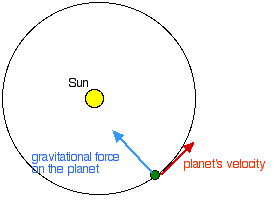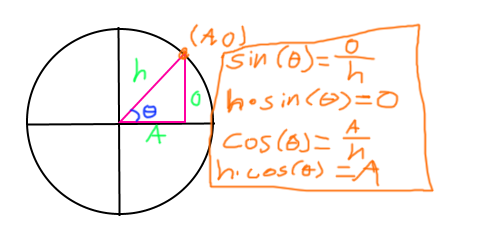# How to Simulate an orbit?

1. What do you want to achieve? Keep it simple and clear!
An average house, just in orbit.

2. What is the issue? Include screenshots / videos if possible!
If the orbit was a straight line, I would have easily tweened it. How would I do it if the path is circular?

3. What solutions have you tried so far? Did you look for solutions on the Developer Hub?
I looked at some body mover properties but they didn’t seem to help. Bezier Curves might help with tweens but I haven’t studied that much so I don’t know quadratic formulas. (we are just learning about y=ax2+mx+b).

I was thinking about using the actual way orbits work in real life. I would have a centripetal force going to the center and a force going to the left, thus creating an
Like this:Personal Opinion

It is in my own opinion that you wait a year or two before trying this, or you start learning more about maths. You stated you are just learning about `y = mx + b` and this is not far enough in the American schooling system at least to have learned any kinematics or trigonometry.

### Option 1: Parameterization of circles

If you just want to just make the given planet go around the object in a circular motion you can use the parameterization of a circle; `x = radius * cos(angle), y = radius * sin(angle)`

This can be derived by looking at the unit circle and some basic trigonometry.Given a right triangle whose “upper most” corner lies on the circle, the hypotenuse is therefore equal to the radius of the circle. Using basic trigonometry we know the `sin(theta) = opposite / hypotenuse)`; rearranging this we can get `hypotenuse * sin(theta) = opposite`. We can apply the same logic to find `adjacent`; `cos(theta) = adjacent / hypotenuse; hypotenuse * cos(theta) = opposite`.

Therefore we can say the location of the “upper most” point on the circle is `(hypotenuse * cos(theta), hypotenuse * sin(theta)`. Abstracting this we can assume `theta` to be the variable of `time`; changing `theta` will now over time construct a circle.

Here is a desmos I set up that you can play with. Where `t` represents time/theta, `a` represents the “time passed” and `b` represents the radius.

### Option 2:

Use formulas that represent this for you!! I’m not too familiar with any of these so I will not indulge into this option, but rather link resources.

Try these articles out.

6 Likes

The trigonometrical formulas look interesting and seem like a good option however the only problem is that I haven’t learnt that much. I will check out the second option and try to comprehend the first option. Thanks for the reply!

This is a complex subject to implement in roblox since it involves things like vector math, trigonometry, and solving the dreaded Kepler’s equation. Here is an old version of how I achieved it:

https://www.roblox.com/library/4829401641/Orbit-Kit

I might come back to this thread tomorrow to explain it a little better.

4 Likes

Have you tried just using a HingeConstraint?
Very simple and easy to set up. My only concern would be the amount of force you are putting on it. However you may be able to just put an opposite BodyVelocity in the house to keep it from trying to blow the HingeConstraint apart.

So basically a hinge so that it revolves?

So simple, it works.

(gotta make up all those dang characters)

If you want to make the house revolve then another hinge at that end.

Basically it uses the exact same formulas that @EpicMetatableMoment mentioned. It just calculates the next point along the circumference of a circle and moves the object there. For roughly simulating an orbit, this is about as simple as it gets.

You could try my module! It is a simple and easy to use free module that contains circular orbits, eccentric orbits and elliptical orbits currently!(I’m updating it soon)!

Get my module here now : Tomroblox54321’s Advanced Planetary Orbit Module!

You could position the house the distance you want away from the center of the object your orbiting then set the pivot point of the house to the center of that object. Then all you’d have to do is rotate it.Just a thought.

Quick Example:

``````local baseplate = game.Workspace.Baseplate
local house = game.Workspace.House

--DO NOT SET A PRIMARY PART
house.WorldPivot = baseplate:GetPivot()

game:GetService("RunService").Heartbeat:Connect(function()
house:PivotTo(house:GetPivot() * CFrame.Angles(0,.01,0))
end)
``````

Its much smoother than in the video… video makes it look glitchy

With a lil extra stuff you can make it simulate an orbit pretty well. If you still need the house model to have a primary part then just wrap the model with another model. Set outermost parent model to not have a primary part so the pivot position will be world position instead of offset.

I’ve done that here and added another rotation to the house itself.

``````local baseplate = game.Workspace.Baseplate
local house = game.Workspace.HouseWrap

--DO NOT SET A PRIMARY PART
house.WorldPivot = baseplate:GetPivot()

game:GetService("RunService").Heartbeat:Connect(function()
house:PivotTo(house:GetPivot() * CFrame.Angles(0,.01,0))
house.House:PivotTo(house.House:GetPivot() * CFrame.Angles(0,-.05,0))
end)
``````